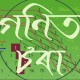## 03 Feb Indian National Mathematical Olympiad (INMO) 2014

The Indian National Mathematical Olympiad (INMO) was held on 2nd February 2014 throughout the country. The test was a four hour duration test, open for high school students who have already qualified the Regional Mathematical Olympiad (RMO). The questions of RMO 2013 can be found here. The questions asked in INMO 2014 are as follows.

1. In a triangle ABC, let D be the point on the segment BC such that AB + BD = AC + CD. Suppose that the points B, C and the centroids of triangles ABD and ACD lie on a circle. Prove that AB = AC.
2. Let n be a natural number. Prove that$\lfloor \frac{n}{1}\rfloor+\lfloor \frac{n}{2}\rfloor+\cdots+\lfloor \frac{n}{n}\rfloor +\lfloor \sqrt{n}\rfloor$ is even.
3. Let a, b be natural numbers with ab > 2. Suppose that the sum of their greatest common divisor and least common multiple is divisble by a + b. Prove that the quotient is at most$\frac{a+b}{4}$. When is this quotient exactly equal to$\frac{a+b}{4}$?
4. Written on a blackboard is the polynomial$x^2 + x + 2014$. Calvin and hobbes take turns alternatively(starting with Calvin) in the following game. During his turns alternatively(starting with Calvin) in the following game. During his turn, Calvin should either increase or decrese the coeffecient of$x$ by 1. And during this turn, Hobbes should either increase or decrease the constant coefficient by 1. Calvin wins if at any point of time the polynomial on the blackboard at that instant has integer roots. Prove that Calvin has a winning stratergy.
5. In a acute-angled triangle ABC, a point D lies on the segment BC. Let$O_1, O_2$ denote the circumcentres of triangles ABD and ACD respectively. Prove that the line joining the circumcentre of triangle ABC and the orthocentre of triangle$O_1O_2D$ is parallel to BC.
6. Let n be a natural number.And let X = {1, 2, …, n} and define A∆B to be the set of all those elements of X which belong to exactly one of A and B.Show that |F| ≤ 2n−1 where F is a collection of subsets of X such that for any two distinct elements of A, B of F,we have |A∆B| ≥ 2.Also find all such collections F for which the maximum is attained.

Download this post as PDF (will not include images and mathematical symbols).

##### Tags:
, ,
•##### cgvgvg
Posted at 17:09h, 09 February Reply

Can one question be cut off for INMO 2014

•##### Gonit Sora
Posted at 12:01h, 21 February Reply

Typically the cutoffs vary from year to year, but it is highly unlikely that one question will be the cutoff.

•##### haer
Posted at 18:18h, 19 February Reply

cutoff 100

•##### cghch
Posted at 12:32h, 21 February Reply

Can 26 marks be the cutoff for INMO 2014?.If not what can be the cutoff.

•##### archit
Posted at 19:16h, 21 February Reply

what is the difficulty of this paper compared to last year and what must be the cut off?